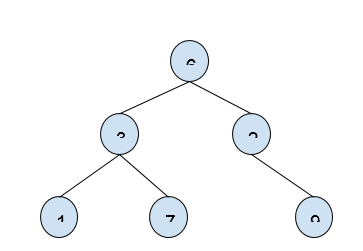# Print BST keys in given Range - O(1) Space in C++

In this problem, we are given two values k1 and k2 (k1 < k2), and the root of the binary search tree. Our task is to create a program to Print BST keys in given Range.

Problem Description: We will print all the keys of the tree from n1 to n2 in increasing order.

Let’s take an example to understand the problem,

Input:k1 = 4, k2 = 12

Output: 6, 7, 9

## Solution Approach

Simple we can solve the problem using inorder traversal but the space complexity there is 0(n) but the need of the hour is to solve in O(1) complexity. So, for this, we will use a special type of traversal technique.

We will use Morris Traversal which is based on threaded binary trees. It does not require any stack/queue and uses NULL pointers to store info, this helps to reduce the storage to O(1).

## Example

Live Demo

#include <iostream>
using namespace std;

struct node {
int data;
struct node *left, *right;
};

node* insertNode(int data) {
node* temp = new node;
temp->data = data;
temp->right = temp->left = NULL;
return temp;
}

void RangeTraversal(node* root, int k1, int k2) {

if (!root)
return;

node* nodeTraversal = root;

while (nodeTraversal) {

if (nodeTraversal->left == NULL) {

if (nodeTraversal->data <= k2 &amp;&amp; nodeTraversal->data >= k1)

cout<<nodeTraversal->data<<" ";
nodeTraversal = nodeTraversal->right;
}

else {
node* prevNode = nodeTraversal->left;
while (prevNode->right != NULL &amp;&amp; prevNode->right != nodeTraversal)
prevNode = prevNode->right;

if (prevNode->right == NULL) {
prevNode->right = nodeTraversal;
nodeTraversal = nodeTraversal->left;
}
else {
prevNode->right = NULL;
if (nodeTraversal->data <= k2 &amp;&amp; nodeTraversal->data >= k1)
cout<<nodeTraversal->data<<" ";
nodeTraversal = nodeTraversal->right;
}
}
}
}

int main() {
node* root = insertNode(6);
root->left = insertNode(3);
root->right = insertNode(2);
root->left->left = insertNode(1);
root->left->right = insertNode(7);
root->right->right = insertNode(9);

cout<<"All BST keys in the given range are \t";
RangeTraversal(root, 4, 10);

return 0;
}

## Output

All BST keys in the given range are 7 6 9